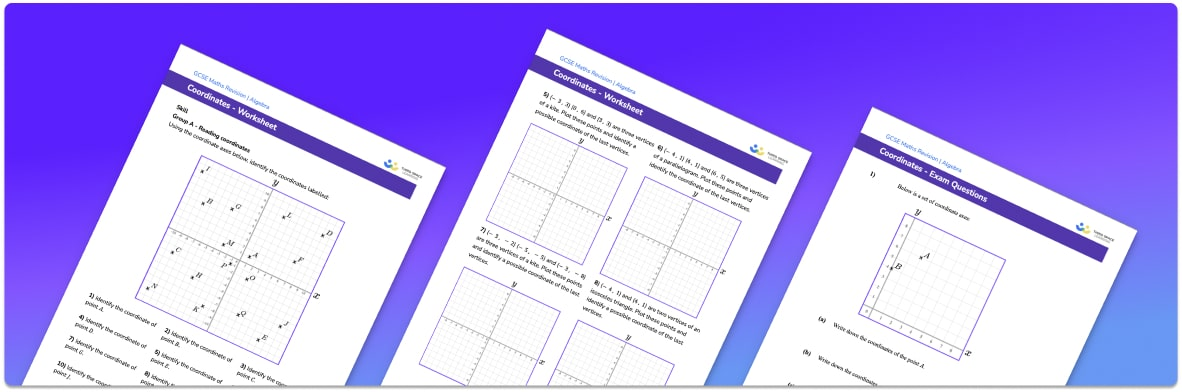# Coordinates Worksheet• Section 1 of the coordinates worksheet contains 20+ skills-based coordinates questions, in 3 groups to support differentiation
• Section 2 of the coordinate plane worksheet contains 3 applied coordinates questions with a mix of worded problems and deeper problem solving questions
• Section 3 contains 3 foundation and higher level GCSE exam style coordinates questions
• Answers and a mark scheme for all geometry worksheets are provided
• Questions follow variation theory with plenty of opportunities for students to work independently at their own level
• All questions created by fully qualified expert secondary maths teachers
• Suitable for GCSE maths revision for AQA, OCR and Edexcel exam boards

• This field is for validation purposes and should be left unchanged.

You can unsubscribe at any time (each email we send will contain an easy way to unsubscribe). To find out more about how we use your data, see our privacy policy.

### Coordinates at a glance

Coordinates are pairs of numbers which identify the position of a point on a coordinate grid. Coordinates of points are given in the form (x, y) where the x coordinate tells you the point’s position on the horizontal axis (the x axis) and the y coordinate tells you the points position on the vertical axis (the y axis). For example, if the point a has coordinates (3, 4), it would line up with 3 on the x axis and 4 on the y axis.

When reading coordinates, we first look at the point’s position in relation to the x axis and write this as the first number in the coordinate pair. We then look at the points position on the y axis and write this as the second number. Coordinates are always written in brackets with a comma between them.

When plotting points, we look at the value of the x coordinate and move along to this position on the x axis. We then look at the y coordinate and move up or down to this position relative to the y axis to plot the point.

Coordinates can be integers or decimals, positive numbers or negative numbers. When introducing how to plot coordinates, it is best to start with coordinates in the first quadrant, with positive values for x and y.

Looking forward, students can then progress to additional types of graphs worksheets and other algebra worksheets, for example a sequences worksheet, simultaneous equations worksheet or straight line graphs worksheet.For more teaching and learning support on Algebra our GCSE maths lessons provide step by step support for all GCSE maths concepts.

## Do you have KS4 students who need more focused attention to succeed at GCSE?There will be students in your class who require individual attention to help them succeed in their maths GCSEs. In a class of 30, it’s not always easy to provide.

Help your students feel confident with exam-style questions and the strategies they’ll need to answer them correctly with our dedicated GCSE maths revision programme.

Lessons are selected to provide support where each student needs it most, and specially-trained GCSE maths tutors adapt the pitch and pace of each lesson. This ensures a personalised revision programme that raises grades and boosts confidence.

Find out more